## Multiplying Polynomials

The following are rules regarding the multiplying of variable expressions.

• Rule 1: To multiply monomials with the same base, keep the base and add the powers:

x ax b = x a + b

• Rule 2: To raise a base to a power, keep the base and multiply the powers.

( x a ) b = x ab

• Rule 3: To raise a product to a power, raise each factor in the product to that power.

( xy) a = x ay a

##### Example 1

Simplify each of the following multiplication problems and state which of the preceding rules was applied.

1. yy 5

2. ( x 4) 3

3. (–2 x 4 y 2 z 3) 5

4. a 3( a 2 b 3) 4

1.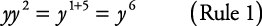2.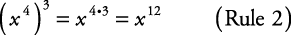3.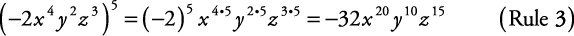4.To multiply monomials together, follow this procedure.

1. Multiply the numerical coefficients together.

2. Multiply the variables together.

3. Write the results as a product.

##### Example 2

Simplify each of the following.

1. (4 x 2)(3 x 3)

2. (–8 a 3 b 2)(2 a 2 b 2) 3

1. (4 x 2)(3 x 3) = (4 × 3)( x 2 x 3) = 12 x 5

2. (–8 a 3 b 2)(2 a 2 b 2) 3 = (–8 a 3 b 2)(8 a 6 b 6) = –64 a 9 b 8

To multiply polynomials together, multiply each term in one polynomial by each term in the other polynomial. Then simplify if possible.

##### Example 3

Multiply each of the following.

1. 5 x(3 x 2 – 4 x + 2)

2. (4 x – 2)(3 x + 5)

3. ( x + y)( x 2xy + y 2)

The following shows how each equation is multiplied both horizontally and vertically.

Equation (a) done horizontally: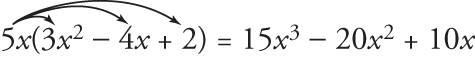Equation (a) done vertically:Equation (b) done horizontally:Equation (b) done vertically:Equation (c) done horizontally: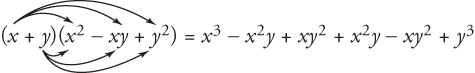Equation (c) done vertically:Back to Top
A18ACD436D5A3997E3DA2573E3FD792A